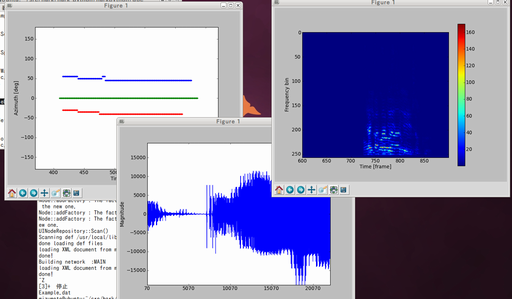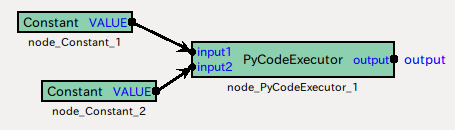# Overview¶

HARK Python is a package that enables Python code execution in HARK written using Boost.Python.

HARK Python provides two functions:

1. Data visualization nodes using matplotlib, a powerful visualization module for python
2. A wrapper to develop a HARK node using python.# Benefits of HARK-Python¶

## Rich visualization¶

The visualization of HARK basic packages is not rich enough. Only DisplayLocalization for visualizing localization results is provided. HARK-Python package provides visualization modules for

• waveform(plotWaveform)
• spectrogram (plotSpec)
• localization(plotSource)

## Quick development of a new node¶

When you use PyCodeExecutor node, you can just write your code. First, write your code like this:

import harkpython.harkbasenode as harkbasenode
class HarkNode (harkbasenode.HarkBaseNode):
def __init__(self):
# define the output names and types of your node as tuples here.
def calculate(self):


Then, build a network that uses your code.

# Installation¶

1. Add HARK repository and install Basic HARK Packages. See HARK installation instructions for details.

2. Install HARK-Python

sudo apt-get install hark-python


# Getting Started¶

## Tutorial 1: Run examples¶

Run the following shell script
/usr/share/doc/hark-python/run_examples.sh


Then, you will see instructions like this:

set the path first:
PYTHONPATH=\$PYTHONPATH:/usr/share/doc/hark-python/examples

Plot localization result
/usr/share/doc/hark-python/examples/plotSourceNetwork.n \
/usr/share/doc/hark-python/examples/plotSourceExample.dat

Plot spectrogram
/usr/share/doc/hark-python/examples/plotSpecNetwork.n \
/usr/share/doc/hark-python/examples/longsample.wav

Plot waveform
/usr/share/doc/hark-python/examples/plotWaveformNetwork.n \
/usr/share/doc/hark-python/examples/shortsample.wav


Following these instructions, set the environmental variable PYTHONPATH first, then, cut and paste the lines.

## Tutorial 2: Implement your node with python¶

The goal is to calculate $$input1^3 + input2^5$$

1. Put two Constants and one PyCodeExecutor into your HARK network, and connect them, then, save as tutorial1.n.1. Set parameters
1. Constant: int 3
2. Constant: int 5
3. PyCodeExecutor: default.
1. Write a code. save it as samplecode.py at the same path as the network file.
import harkpython.harkbasenode as harkbasenode

class HarkNode(harkbasenode.HarkBaseNode):
def __init__(self):
self.outputNames=("output",)  # one output terminal named "output"
self.outputTypes=("prim_float",)  # the type is primitive float.

def calculate(self):
self.outputValues["output"] = self.input1 ** 3 + self.input2 ** 5
# set output value
# from two inputs: input1 and input2.

1. Run

./tutorial1.n


## Tutorial 3: Simple container examples¶

[TODO] Use Vector, Matrix, and Map.

# Description¶

## harkbasenode¶

harkbasenode is a base class for HARK Python that interfaces the python code and HARK processing. To write a code for HARK Python, you MUST inherit the harkbasenode class.

For details, see /usr/share/doc/hark-python/harkbasenode.html or in python interpreter, run

import harkpython.harkbasenode
help(harkpython.harkbasenode)


## Support types¶

Primitives
int, float, complex<float>, and ObjectRef (= Source)
Containers
Vector<T> [T = int, float, complex<float>, and Source] Matrix<T> [T = int, float, complex<float>] Map<int, T> [T = Vector<int>, Vector<float>, Vector<complex<float> >, Float, Matrix<int>, Matrix<float>, Matrix<complex<float> >]

## Instance variables¶

self.nodeName
The name of the node. e.g., node_PyCodeExecutor_1.
self.nodeID
The ID of the node. if the node name is node_PyCodeExecutor_1, the ID is 1.
self.count
The number of iterations.

If you want to plot the data for every 100 frames,

if self.count % 100 == 0
pylab.plot(data)
pylab.draw()


## Parameters of PyCodeExecuter and how they are used¶

DIRECTORY_NAME
This path is added to python path.
FILENAME
The file name of your code. No extensions.
CLASSNAME
The class name in your code that will be instanciated.

Equivalent code is:

import sys
sys.path.append(DIRECTORY_NAME)
import FILENAME
object = FILENAME.CLASSNAME()

# For each iteration, calculate() is called
object.calculate()


# Tips¶

## Can I add a parameter to the script?¶

Currently, PyCodeExecutor does not pass the given parameters to the scripts. Instead, since PyCodeExecutor can receive an arbitrary number of inputs, you can realize this using a Constant node.

For example, if you want to add a subnet_param named THRESHOLD to your PyCodeExecutor,

1. Add THRESHOLD input to PyCodeExecutor.
2. Add Constant node and connect its output to THRESHOLD.
3. Change the type of the VALUE of the Constant node to subnet_param.

## Can I improve the calculation speed?¶

Some tips exist to improve processing speed.

1. Use python packages to increase speed: For example, using numpy or scipy will improve the scientific computation including matrix calculation, cython for using C functions, or scipy.weave for embedding C++ code into your python code.

2. skip calculation For example, plotting is too heavy to execute at every frame (i.e., 10 msec for default setting). In that case, you can use an instance variable self.count to execute the function at a regular interval.

Here is an example code:

def calculate(self):
if not self.count % 100 == 0:
break

# do some heavy_calculation


However, if the requirement of calculation speed is really high, you should use HARK-Python only for prototyping, and write the code in C++, which is the standard way in HARK.

## I want to see other python code examples¶

HARK Python includes some examples as described in tutorial 1. The python codes are installed in /usr/lib/python2.7/dist-packages/harkpython.